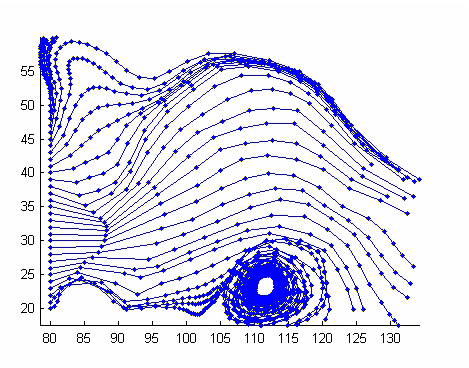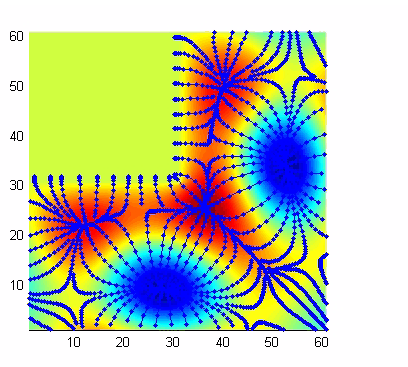MATLAB Function Referenceinterpstreamspeed

Interpolate stream line vertices from flow speed

Syntax

• ```interpstreamspeed(X,Y,Z,U,V,W,vertices)
interpstreamspeed(U,V,W,vertices)
interpstreamspeed(X,Y,Z,speed,vertices)
interpstreamspeed(speed,vertices)

interpstreamspeed(X,Y,U,V,vertices)
interpstreamspeed(U,V,vertices)
interpstreamspeed(X,Y,speed,vertices)
interpstreamspeed(speed,vertices)

interpstreamspeed(...,sf)
vertsout = interpstreamspeed(...)
```

Description

```interpstreamspeed(X,Y,Z,U,V,W,vertices) ``` interpolates streamline vertices based on the magnitude of the vector data `U`, `V`, `W`. The arrays `X`, `Y`, `Z` define the coordinates for `U`, `V`, `W` and must be monotonic and 3-D plaid (as if produced by `meshgrid`).

```interpstreamspeed(U,V,W,vertices) ``` assumes `X`, `Y`, and `Z` are determined by the expression

• ```[X Y Z] = meshgrid(1:n,1:m,1:p)
```

where `[m n p] = size(U)`.

```interpstreamspeed(X,Y,Z,speed,vertices) ``` uses the 3-D array `speed` for the speed of the vector field.

```interpstreamspeed(speed,vertices) ``` assumes `X`, `Y`, and `Z` are determined by the expression

• ```[X Y Z] = meshgrid(1:n,1:m,1:p)
```

where `[m n p]=size(speed)`.

```interpstreamspeed(X,Y,U,V,vertices) ``` interpolates streamline vertices based on the magnitude of the vector data `U`, `V`. The arrays `X`, `Y` define the coordinates for `U`, `V` and must be monotonic and 2-D plaid (as if produced by `meshgrid`)

```interpstreamspeed(U,V,vertices) ``` assumes `X` and `Y` are determined by the expression

• ```[```X Y] = meshgrid(1:n,1:m)
``````

where `[M N]=size(U)`.

```interpstreamspeed(X,Y,speed,vertices) ``` uses the 2-D array `speed` for the speed of the vector field.

```interpstreamspeed(speed,vertices) ``` assumes `X` and `Y` are determined by the expression

• ```[X Y] = meshgrid(1:n,1:m)
```

where `[M,N]= size(speed)`.

```interpstreamspeed(...,sf) ``` uses `sf` to scale the magnitude of the vector data and therefore controls the number of interpolated vertices. For example, if `sf` is 3, then `interpstreamspeed` creates only one-third of the vertices.

```vertsout = interpstreamspeed(...) ``` returns a cell array of vertex arrays.

Examples

This example draws streamlines using the vertices returned by `interpstreamspeed`. Dot markers indicate the location of each vertex. This example enables you to visualize the relative speeds of the flow data. Streamlines having widely spaced vertices indicate faster flow; those with closely spaced vertices indicate slower flow.

• ```load wind
[sx sy sz] = meshgrid(80,20:1:55,5);
verts = stream3(x,y,z,u,v,w,sx,sy,sz);
iverts = interpstreamspeed(x,y,z,u,v,w,verts,.2);
sl = streamline(iverts);
set(sl,'Marker','.')
axis tight; view(2); daspect([1 1 1])```

This example plots streamlines whose vertex spacing indicates the value of the gradient along the streamline.

• ```z = membrane(6,30);
[u v] = gradient(z);
[verts averts] = streamslice(u,v);
iverts = interpstreamspeed(u,v,verts,15);
sl = streamline(iverts);
set(sl,'Marker','.')
hold on; pcolor(z); shading interp
axis tight; view(2); daspect([1 1 1])```

See Also

`stream2`, `stream3`, `streamline`, `streamslice`, `streamparticles`

Volume Visualization for related functions

© 1994-2005 The MathWorks, Inc.